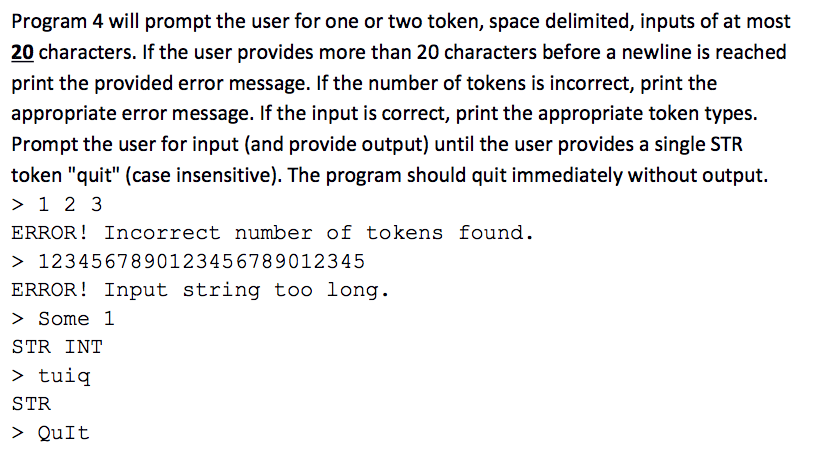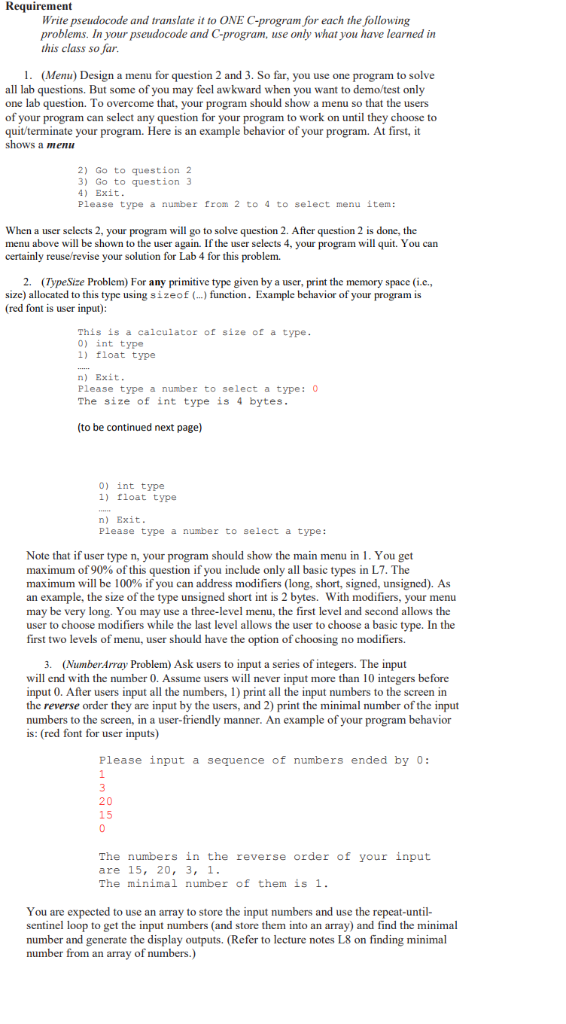Question

Design pseudocode for a program that accepts a single number from the user then outputs one of the following message as appropriate: The number is larger than 0, The number is smaller than 0, The number is 0. HINTS AND NOTES: Be sure to prompt the user for all input.

Pseudocode:

Step 1: Start the program

Step 2: Prompt the user to enter a number and store it in number.

Step 3: Check if the number is greater than 0 (if number > 0), then

Step 4: print to the screen, "The number is larger than 0"

Step 5: Else if the number is less than 0 (else if number < 0), then

Step 6: print to the screen, "The number is smaller than 0"

Step 7: Else print to the screen, "The number is 0"

Step 8: End the program.

Hope this helps!

#### Earn Coins

Coins can be redeemed for fabulous gifts.

Similar Homework Help Questions
• ### Create a design document for a program that will take a number from the user and...

Create a design document for a program that will take a number from the user and convert it to IEEE single precision format. The program displays the IEEE representation (Single precision) of the number entered by the user. PLEASE ADD THE PSEUDOCODE AND CODE WITH C PROGRAMMING

• ### this assignment should be delivered in C language Program 4 will prompt the user for one...this assignment should be delivered in C language Program 4 will prompt the user for one or two token, space delimited, inputs of at most 20 characters. If the user provides more than 20 characters before a newline is reached print the provided error message. If the number of tokens is incorrect, print the appropriate error message. If the input is correct, print the appropriate token types. Prompt the user for input (and provide output) until the user provides a...

• ### Design a calculator program that will add, subtract, multiply, or divide two numbers input by a user.

Design a calculator program that will add, subtract, multiply, or divide two numbers input by a user.Your program design should contain the following:The main menu of your program is to continue to prompt the user for an arithmetic choice until the user enters a sentinel value to quit the calculator program.When the user chooses an arithmetic operation (i.e. addition) the operation is to continue to be performed (i.e. prompting the user for each number, displaying theresult, prompting the user to...

• ### Design a program in pseudocode that asks the user to enter a string containing a series...

Design a program in pseudocode that asks the user to enter a string containing a series of ten single-digit numbers with nothing separating them. The program should display the sum of all the single digit numbers in the string except for the highest value digit. You may only use one main module and one sorting function; otherwise, do not modularize your program. For example, if the user enters 5612286470, the sum would be 33. (0+1+2+2+4+5+6+6+7) If there are multiple highest...

• ### In C:Write a program that can determine whether a user input is a binary number or...

In C:Write a program that can determine whether a user input is a binary number or not. (1, 2, 3, 8, 13) Write a program that accomplishes all of the following: Greets the user and informs them that the program will determine whether an input from the user is a valid binary number or not (e.g., consisting of only 0’s and/or 1’s). Accept an integer input from the user. Assume that the user provides a valid numeric input that will...

• ### 2. Write a Marie program that accepts two positive (inputs) two integers and outputs the sum...

2. Write a Marie program that accepts two positive (inputs) two integers and outputs the sum of both of the integers and all the numbers in between Write a Marie program that determines the largest of a series of positive integers provided by a user. The user will enter a -1 when she is finished. Up to 10 numbers will be provided by the user. Write a Marie program that accepts two positive integers, multiples them by repeated addition, and...

• ### Prepare pseudocode for a program that lets a user continuously enter numbers until the number 99...

Prepare pseudocode for a program that lets a user continuously enter numbers until the number 99 is entered. Once the user has stopped entering numbers, display the number of numbers entered AND the sum of those numbers. You'll need a counter and an accumulator for this (see pp. 179-181 and 205-208). Make sure your loop is structured (see priming input in figure 3-16). Don't forget to comment your code. Don't forget to display appropriate prompts when requesting input from the...

• ### Write a program in Python that accepts as input an integer N and a real number...

Write a program in Python that accepts as input an integer N and a real number c, and outputs the coefficient of the Nth degree term of the Taylor series for the function f(x) = ex centered at c. This process should recur until the user enters a quit code. Erroneous input should be excepted as necessary, and should result in a new prompt.

• ### Design and implement a Java program (name it PasswordTest) that accepts a string from the user...Design and implement a Java program (name it PasswordTest) that accepts a string from the user and evaluates it as a password, giving it a valid or invalid verdict A good password will be at least 8 characters and includes at least one lower-case letter, at least one upper-case letter, at least one digit, and at least one character that is neither a letter nor a digit. Your program will need to check each character in the string in order...

• ### Requirement Write pseudocode and translate it to ONE C-program for each the following problems. In your...Requirement Write pseudocode and translate it to ONE C-program for each the following problems. In your pseudocode and C-program, use only what you have learned in this class so far. (Menu) Design a menu for question 2 and 3. So far, you use one program to solve all lab questions. But some of you may feel awkward when you want to demo/test only one lab question. To overcome that, your program should show a menu so that the users of...